# FE Review Engineering Economics.pdf

Apr. 2, 2023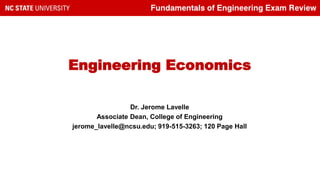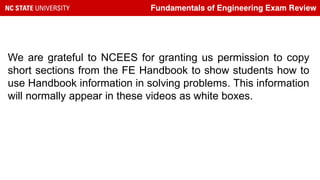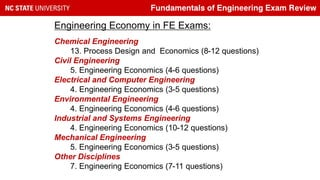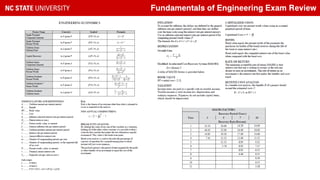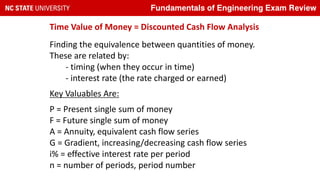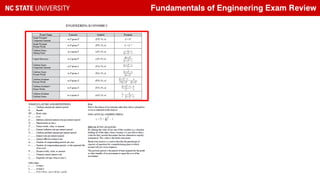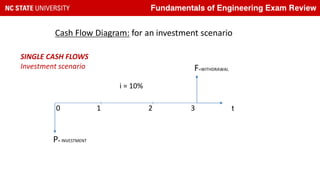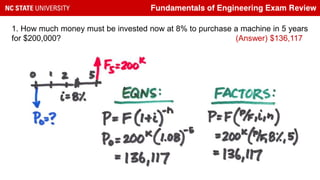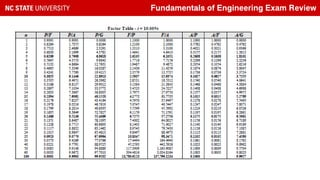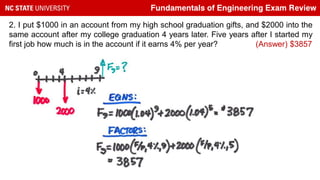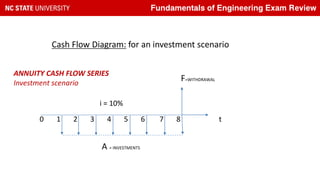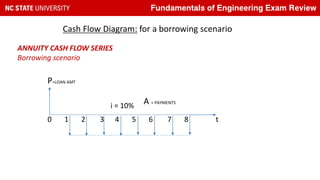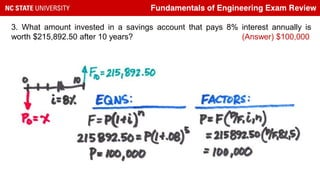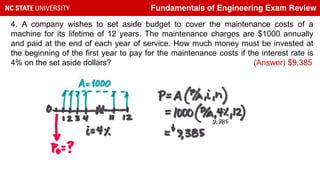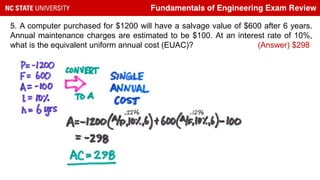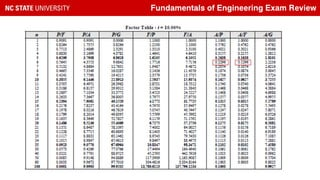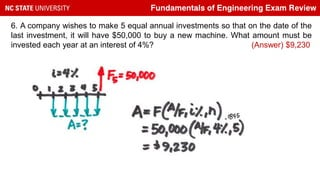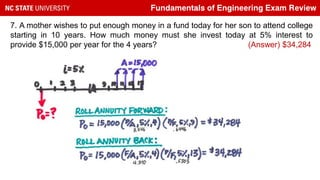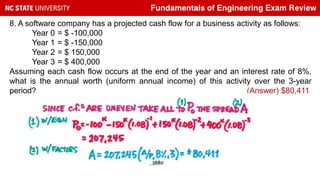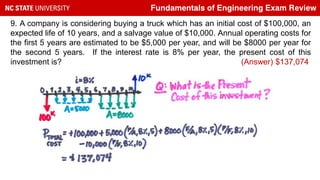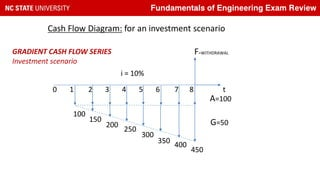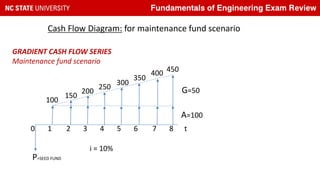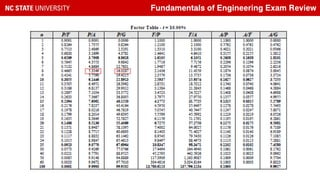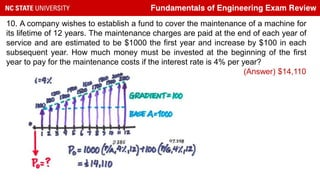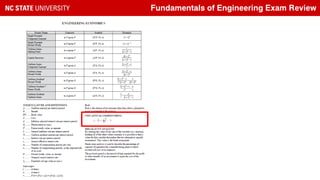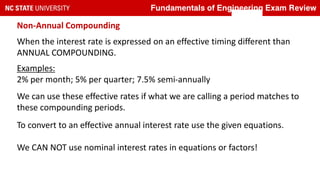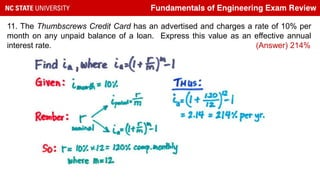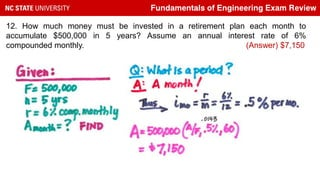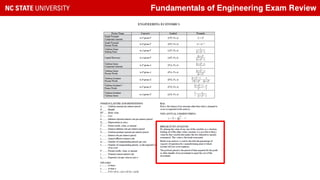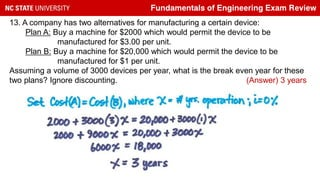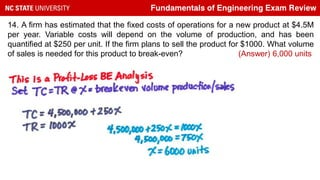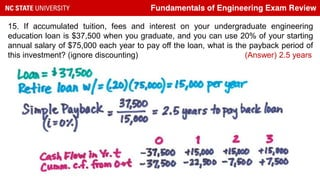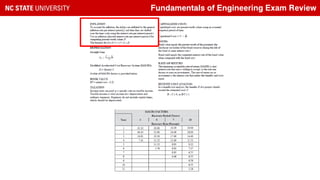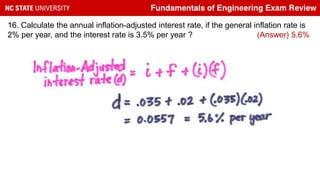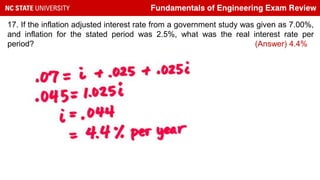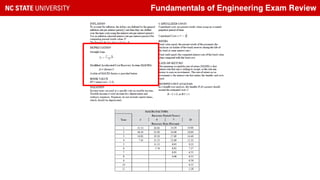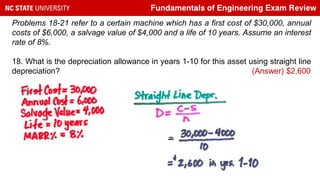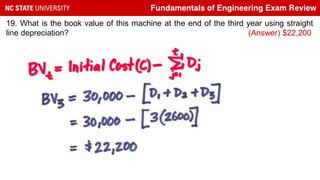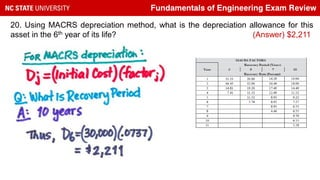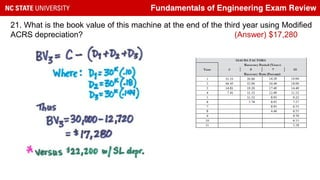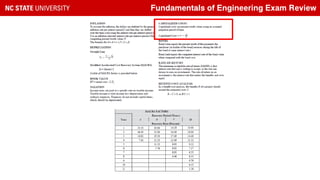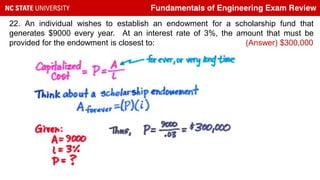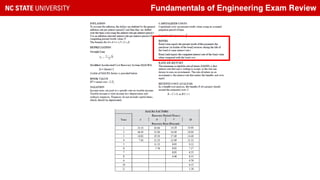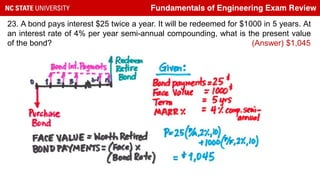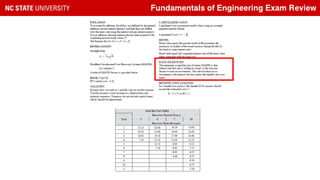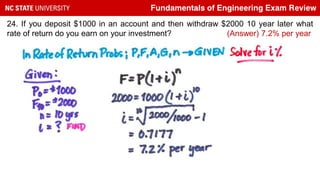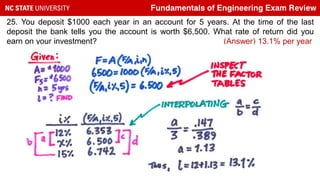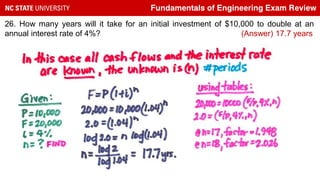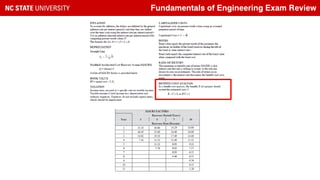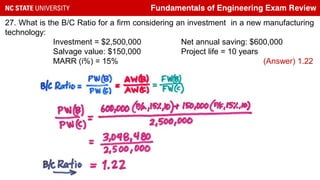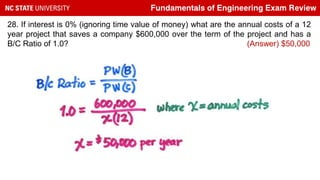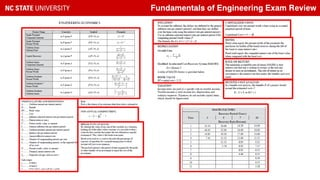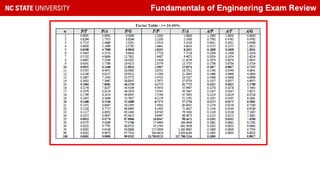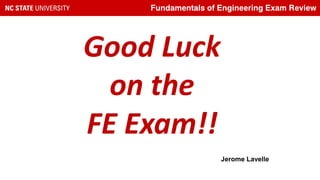1 of 54

### FE Review Engineering Economics.pdf

1. Fundamentals of Engineering Exam Review Engineering Economics Dr. Jerome Lavelle Associate Dean, College of Engineering jerome_lavelle@ncsu.edu; 919-515-3263; 120 Page Hall
2. Fundamentals of Engineering Exam Review We are grateful to NCEES for granting us permission to copy short sections from the FE Handbook to show students how to use Handbook information in solving problems. This information will normally appear in these videos as white boxes.
3. Fundamentals of Engineering Exam Review Engineering Economy in FE Exams: Chemical Engineering 13. Process Design and Economics (8-12 questions) Civil Engineering 5. Engineering Economics (4-6 questions) Electrical and Computer Engineering 4. Engineering Economics (3-5 questions) Environmental Engineering 4. Engineering Economics (4-6 questions) Industrial and Systems Engineering 4. Engineering Economics (10-12 questions) Mechanical Engineering 5. Engineering Economics (3-5 questions) Other Disciplines 7. Engineering Economics (7-11 questions)
4. Fundamentals of Engineering Exam Review
5. Fundamentals of Engineering Exam Review Time Value of Money = Discounted Cash Flow Analysis Finding the equivalence between quantities of money. These are related by: - timing (when they occur in time) - interest rate (the rate charged or earned) Key Valuables Are: P = Present single sum of money F = Future single sum of money A = Annuity, equivalent cash flow series G = Gradient, increasing/decreasing cash flow series i% = effective interest rate per period n = number of periods, period number
6. Fundamentals of Engineering Exam Review
7. Fundamentals of Engineering Exam Review Cash Flow Diagram: for an investment scenario SINGLE CASH FLOWS Investment scenario 0 1 2 3 t P= INVESTMENT F=WITHDRAWAL i = 10%
8. Fundamentals of Engineering Exam Review 1. How much money must be invested now at 8% to purchase a machine in 5 years for \$200,000? (Answer) \$136,117
9. Fundamentals of Engineering Exam Review
10. Fundamentals of Engineering Exam Review 2. I put \$1000 in an account from my high school graduation gifts, and \$2000 into the same account after my college graduation 4 years later. Five years after I started my first job how much is in the account if it earns 4% per year? (Answer) \$3857
11. Fundamentals of Engineering Exam Review Cash Flow Diagram: for an investment scenario ANNUITY CASH FLOW SERIES Investment scenario 0 1 2 3 4 5 6 7 8 t A = INVESTMENTS F=WITHDRAWAL i = 10%
12. Fundamentals of Engineering Exam Review Cash Flow Diagram: for a borrowing scenario ANNUITY CASH FLOW SERIES Borrowing scenario A = PAYMENTS P=LOAN AMT 0 1 2 3 4 5 6 7 8 t i = 10%
13. Fundamentals of Engineering Exam Review 3. What amount invested in a savings account that pays 8% interest annually is worth \$215,892.50 after 10 years? (Answer) \$100,000
14. Fundamentals of Engineering Exam Review 4. A company wishes to set aside budget to cover the maintenance costs of a machine for its lifetime of 12 years. The maintenance charges are \$1000 annually and paid at the end of each year of service. How much money must be invested at the beginning of the first year to pay for the maintenance costs if the interest rate is 4% on the set aside dollars? (Answer) \$9,385
15. Fundamentals of Engineering Exam Review 5. A computer purchased for \$1200 will have a salvage value of \$600 after 6 years. Annual maintenance charges are estimated to be \$100. At an interest rate of 10%, what is the equivalent uniform annual cost (EUAC)? (Answer) \$298
16. Fundamentals of Engineering Exam Review
17. Fundamentals of Engineering Exam Review 6. A company wishes to make 5 equal annual investments so that on the date of the last investment, it will have \$50,000 to buy a new machine. What amount must be invested each year at an interest of 4%? (Answer) \$9,230
18. Fundamentals of Engineering Exam Review 7. A mother wishes to put enough money in a fund today for her son to attend college starting in 10 years. How much money must she invest today at 5% interest to provide \$15,000 per year for the 4 years? (Answer) \$34,284
19. Fundamentals of Engineering Exam Review 8. A software company has a projected cash flow for a business activity as follows: Year 0 = \$ -100,000 Year 1 = \$ -150,000 Year 2 = \$ 150,000 Year 3 = \$ 400,000 Assuming each cash flow occurs at the end of the year and an interest rate of 8%, what is the annual worth (uniform annual income) of this activity over the 3-year period? (Answer) \$80,411
20. Fundamentals of Engineering Exam Review 9. A company is considering buying a truck which has an initial cost of \$100,000, an expected life of 10 years, and a salvage value of \$10,000. Annual operating costs for the first 5 years are estimated to be \$5,000 per year, and will be \$8000 per year for the second 5 years. If the interest rate is 8% per year, the present cost of this investment is? (Answer) \$137,074
21. Fundamentals of Engineering Exam Review Cash Flow Diagram: for an investment scenario GRADIENT CASH FLOW SERIES Investment scenario 0 1 2 3 4 5 6 7 8 t A=100 F=WITHDRAWAL i = 10% G=50 100 150 200 250 300 350 400 450
22. Fundamentals of Engineering Exam Review Cash Flow Diagram: for maintenance fund scenario GRADIENT CASH FLOW SERIES Maintenance fund scenario 0 1 2 3 4 5 6 7 8 t A=100 P=SEED FUND i = 10% G=50 100 150 200 250 300 350 400 450
23. Fundamentals of Engineering Exam Review
24. Fundamentals of Engineering Exam Review 10. A company wishes to establish a fund to cover the maintenance of a machine for its lifetime of 12 years. The maintenance charges are paid at the end of each year of service and are estimated to be \$1000 the first year and increase by \$100 in each subsequent year. How much money must be invested at the beginning of the first year to pay for the maintenance costs if the interest rate is 4% per year? (Answer) \$14,110
25. Fundamentals of Engineering Exam Review
26. Fundamentals of Engineering Exam Review Non-Annual Compounding When the interest rate is expressed on an effective timing different than ANNUAL COMPOUNDING. Examples: 2% per month; 5% per quarter; 7.5% semi-annually We can use these effective rates if what we are calling a period matches to these compounding periods. To convert to an effective annual interest rate use the given equations. We CAN NOT use nominal interest rates in equations or factors!
27. Fundamentals of Engineering Exam Review 11. The Thumbscrews Credit Card has an advertised and charges a rate of 10% per month on any unpaid balance of a loan. Express this value as an effective annual interest rate. (Answer) 214%
28. Fundamentals of Engineering Exam Review 12. How much money must be invested in a retirement plan each month to accumulate \$500,000 in 5 years? Assume an annual interest rate of 6% compounded monthly. (Answer) \$7,150
29. Fundamentals of Engineering Exam Review
30. Fundamentals of Engineering Exam Review 13. A company has two alternatives for manufacturing a certain device: Plan A: Buy a machine for \$2000 which would permit the device to be manufactured for \$3.00 per unit. Plan B: Buy a machine for \$20,000 which would permit the device to be manufactured for \$1 per unit. Assuming a volume of 3000 devices per year, what is the break even year for these two plans? Ignore discounting. (Answer) 3 years
31. Fundamentals of Engineering Exam Review 14. A firm has estimated that the fixed costs of operations for a new product at \$4.5M per year. Variable costs will depend on the volume of production, and has been quantified at \$250 per unit. If the firm plans to sell the product for \$1000. What volume of sales is needed for this product to break-even? (Answer) 6,000 units
32. Fundamentals of Engineering Exam Review 15. If accumulated tuition, fees and interest on your undergraduate engineering education loan is \$37,500 when you graduate, and you can use 20% of your starting annual salary of \$75,000 each year to pay off the loan, what is the payback period of this investment? (ignore discounting) (Answer) 2.5 years
33. Fundamentals of Engineering Exam Review
34. Fundamentals of Engineering Exam Review 16. Calculate the annual inflation-adjusted interest rate, if the general inflation rate is 2% per year, and the interest rate is 3.5% per year ? (Answer) 5.6%
35. Fundamentals of Engineering Exam Review 17. If the inflation adjusted interest rate from a government study was given as 7.00%, and inflation for the stated period was 2.5%, what was the real interest rate per period? (Answer) 4.4%
36. Fundamentals of Engineering Exam Review
37. Fundamentals of Engineering Exam Review Problems 18-21 refer to a certain machine which has a first cost of \$30,000, annual costs of \$6,000, a salvage value of \$4,000 and a life of 10 years. Assume an interest rate of 8%. 18. What is the depreciation allowance in years 1-10 for this asset using straight line depreciation? (Answer) \$2,600
38. Fundamentals of Engineering Exam Review 19. What is the book value of this machine at the end of the third year using straight line depreciation? (Answer) \$22,200
39. Fundamentals of Engineering Exam Review 20. Using MACRS depreciation method, what is the depreciation allowance for this asset in the 6th year of its life? (Answer) \$2,211
40. Fundamentals of Engineering Exam Review 21. What is the book value of this machine at the end of the third year using Modified ACRS depreciation? (Answer) \$17,280
41. Fundamentals of Engineering Exam Review
42. Fundamentals of Engineering Exam Review 22. An individual wishes to establish an endowment for a scholarship fund that generates \$9000 every year. At an interest rate of 3%, the amount that must be provided for the endowment is closest to: (Answer) \$300,000
43. Fundamentals of Engineering Exam Review
44. Fundamentals of Engineering Exam Review 23. A bond pays interest \$25 twice a year. It will be redeemed for \$1000 in 5 years. At an interest rate of 4% per year semi-annual compounding, what is the present value of the bond? (Answer) \$1,045
45. Fundamentals of Engineering Exam Review
46. Fundamentals of Engineering Exam Review 24. If you deposit \$1000 in an account and then withdraw \$2000 10 year later what rate of return do you earn on your investment? (Answer) 7.2% per year
47. Fundamentals of Engineering Exam Review 25. You deposit \$1000 each year in an account for 5 years. At the time of the last deposit the bank tells you the account is worth \$6,500. What rate of return did you earn on your investment? (Answer) 13.1% per year
48. Fundamentals of Engineering Exam Review 26. How many years will it take for an initial investment of \$10,000 to double at an annual interest rate of 4%? (Answer) 17.7 years
49. Fundamentals of Engineering Exam Review
50. Fundamentals of Engineering Exam Review 27. What is the B/C Ratio for a firm considering an investment in a new manufacturing technology: Investment = \$2,500,000 Net annual saving: \$600,000 Salvage value: \$150,000 Project life = 10 years MARR (i%) = 15% (Answer) 1.22
51. Fundamentals of Engineering Exam Review 28. If interest is 0% (ignoring time value of money) what are the annual costs of a 12 year project that saves a company \$600,000 over the term of the project and has a B/C Ratio of 1.0? (Answer) \$50,000
52. Fundamentals of Engineering Exam Review
53. Fundamentals of Engineering Exam Review
54. Fundamentals of Engineering Exam Review Good Luck on the FE Exam!! Jerome Lavelle